## Example Questions

← Previous 1

### Example Question #1 : Sectors

John owns 8 black shirts, 7 red shirts, 6 blue shirts and 4 white shirts.  If he wants to make a circle chart of his shirts, what is the degree angle corresponding to the "blue shirt section?"

25.0 degrees

The answer cannot be determined from the above information.

90 degrees

43.2 degrees

86.4 degrees

86.4 degrees

Explanation:

We can set up a ratio to calculate the angle measure as such: 6/25 = x/360, since there are 360 degrees in a circle.  Solving, we obtain x = 86.4 degrees.

### Example Question #29 : Geometry

A circular pie is cut into 30 pieces. Two people wish to split a piece of the pie, but one person wants to have twice as much as the other person. What is the angle of the smaller piece produced in this manner?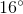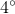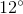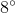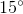Explanation:

First of all, calculate the angle of each of the full pieces of pie. This is easily found: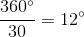Though small, this is what the information tells us! Now, we know that if two people are eating the piece, with one having twice the amount of the other, the angles must befor the smaller piece andfor the larger one. Thus, we can write the equation: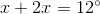Simplifying, we get: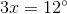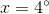That is a tiny piece, but that is what is called for by the data!

### Example Question #30 : Geometry

Quantity A: The angle of a circle's sector having an arc length of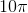and a radius of.

Quantity B: The angle of a circle's sector having an area of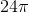and a radius of.

Which of the following relations is true?

The two quantities are equal.

Quantity A is larger than quantity B.

The relationship between the two values cannot be determined.

Quantity B is larger than quantity A.

Quantity A is larger than quantity B.

Explanation:

For each of these, you need to compute the total measurement applicable to the given data. For Quantity A, this will be the total circumference. For Quantity B, this will be the total area. You will then divide the given sector calculation by this total amount. By multiplying this percentage by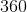, you will find the degree measure of each; however, you will merely need to stop at the percentage (since both are percentages of the same number, namely).

Quantity A

The total circumference is calculated using the standard equation:or, for our data: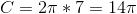Thus, our pertinent percentage is: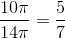Quantity B

For this, the area is computed by the formula:or, for our data: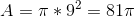Thus, our percentage is: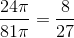Clearly,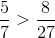, so A is larger than B.

### Example Question #31 : Geometry

What is the perimeter of a pie piece if the pie is sliced into 40 degree pieces and its area is 361π?

40.1π

2.1

38π/9

38π

38 + 38π/9

38 + 38π/9

Explanation:

The perimeter of a given pie-piece will be equal to 2 radii plus the outer arc (which is a percentage of the circumference). For our piece, this arc will be 40/360 or 1/9 of the circumference. If the area is 361π, this means πr2 = 361 and that the radius is 19. The total circumference is therefore 2 * 19 * π or 38π.

The total perimeter of the pie piece is therefore 2 * r + (1 / 9) * c = 2 * 19 + (1/9) * 38π = 38 + 38π/9

### Example Question #32 : Geometry

What is the length of the arc of a circle with radius 10 that traces a 50 degree angle?

25π

25π/29

20π/9

25π/7

25π/9

25π/9

Explanation:

length of an arc = (degrees * 2πr)/360 = (50 * 2π * 10)/360 = 25π/9

### Example Question #33 : Geometry

An ant walks around the edge of circular pizzas left on the counter of a pizza shop. On most days, it is shaken off the pizza before it manages to walk the complete distance.

Quantity A: The distance covered by the ant when walking over four slices of a pizza with a diameter ofandequally-sized pieces.

Quantity B: The distance covered by the ant when walking over a complete personal pizza with a diameter ofinches.

What can we say about the two quantities?

The relationship between the two quantities cannot be determined.

Quantity B is larger.

Quantity A is larger.

The two quantities are equal.

Quantity B is larger.

Explanation:

Let's compute each quantity.

Quantity A

This is a little bit more difficult than quantity B.  It requires us to compute an arc length, for the ant does not walk around the whole pizza; however, this is not very hard. What we know is that the ant walks around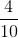, or, of the pizza.

Now, we know the circumference of a circle isor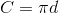For our example, let's use the latter. Since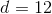, we know: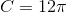However, our ant walks around only part of this, namely: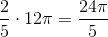Quantity B

This is really just a matter of computing the circumference of the circle.  For our value, we know this to be: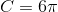Now, we can compare these by taking quantity A and reducing the fraction to be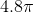. Thus, we know that Quantity B is larger than Quantity A.

### Example Question #1 : How To Find The Percentage Of A Sector From An Angle

A circle of radius, for an angle, has an arc length of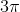. What is the angle?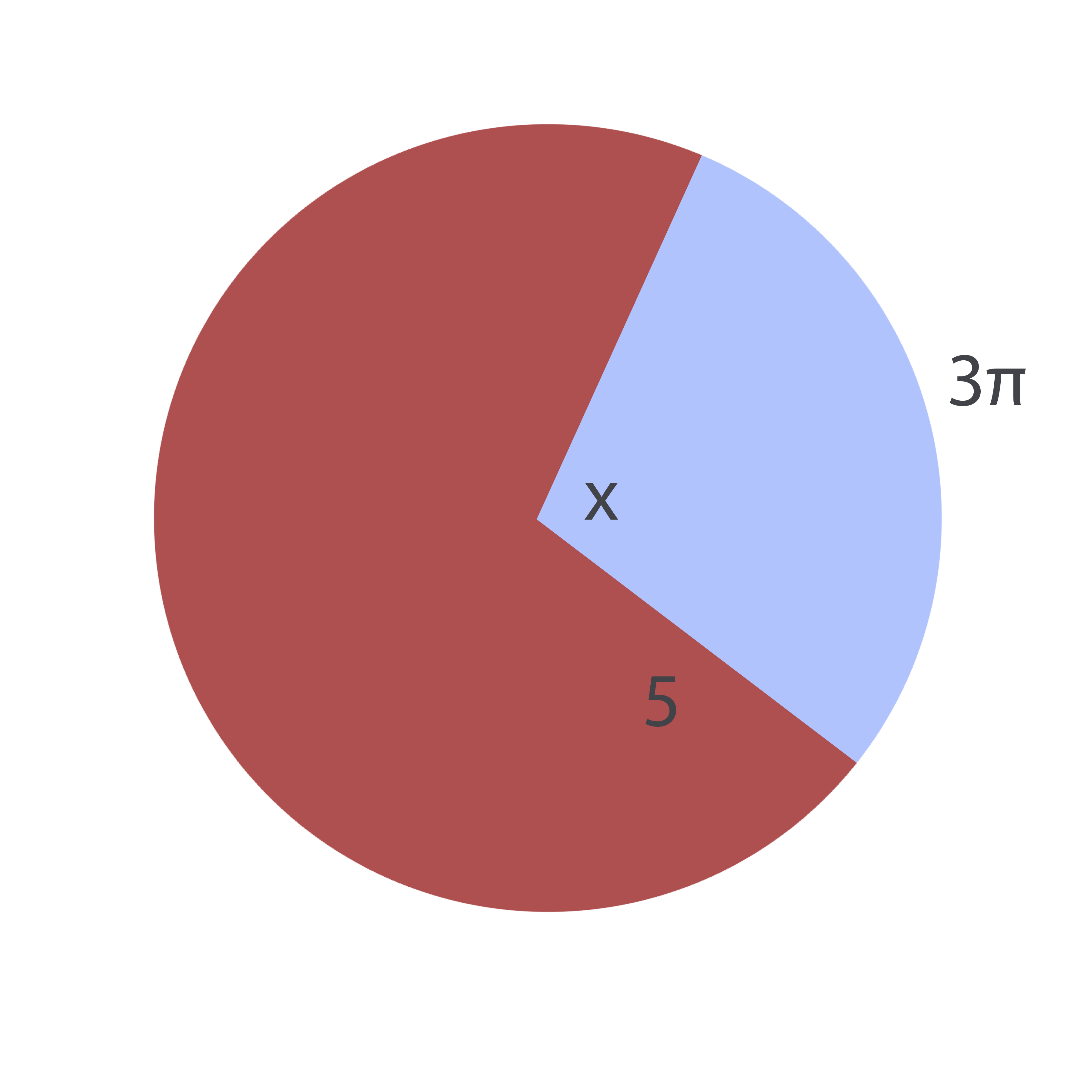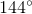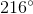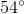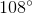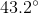Explanation:

The formula for an arc length,, of a circle of a given radius,, and a given angle,is: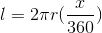Note that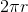is the circumference of the circle.

Conversely, for a known arc length and unknown angle, this equation can be rewritten as follows: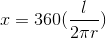Plugging in the given values, it is therefore possible to find the missing angle: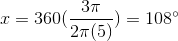### Example Question #2 : How To Find The Percentage Of A Sector From An Angle

Find the radius of the given circle: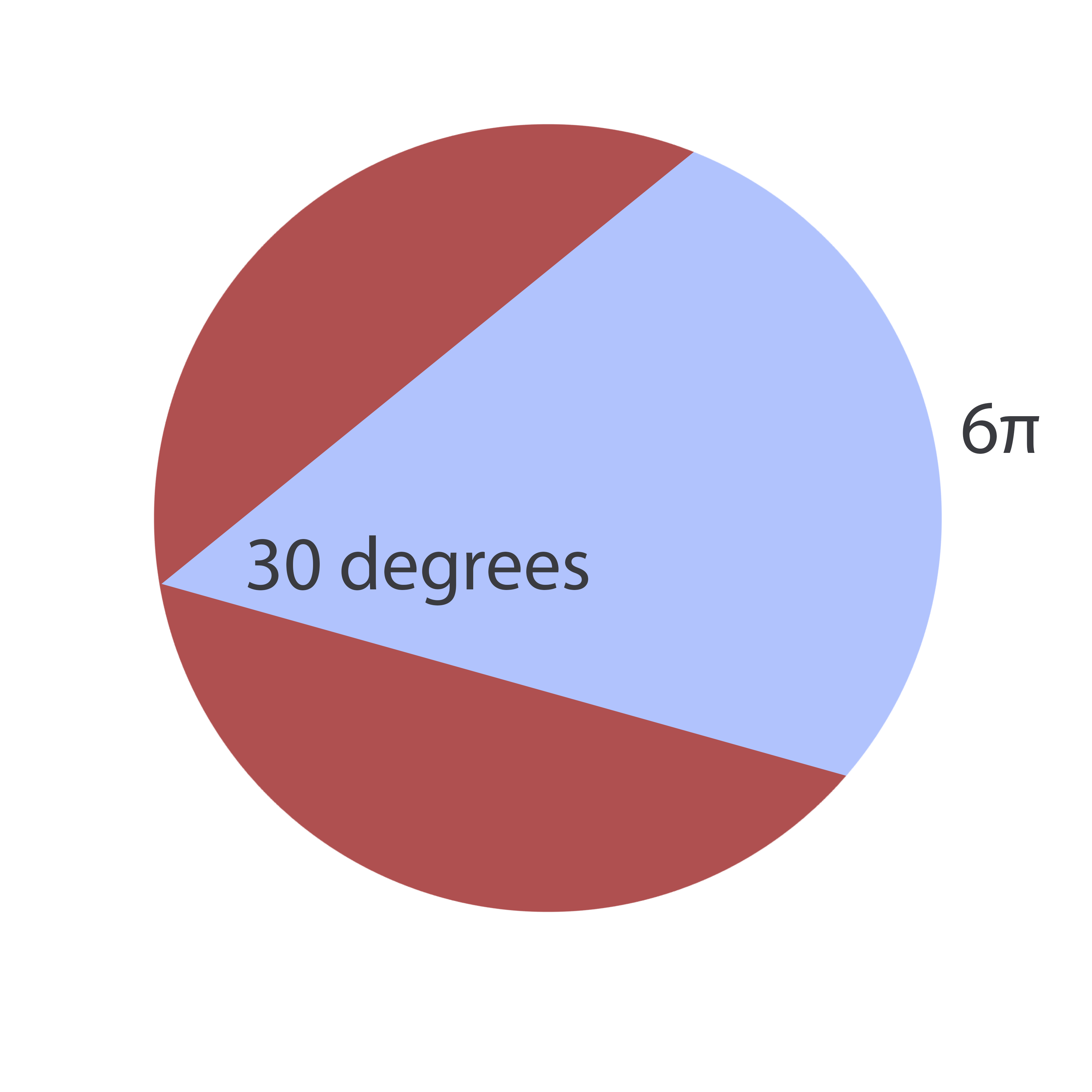The answer cannot be determined from the information given.Explanation:

To solve this problem, realize that an inscribed angle (an angle formed by two chords) is equal to twice the central angle formed by connecting the origin to the inscribed angle's endpoints: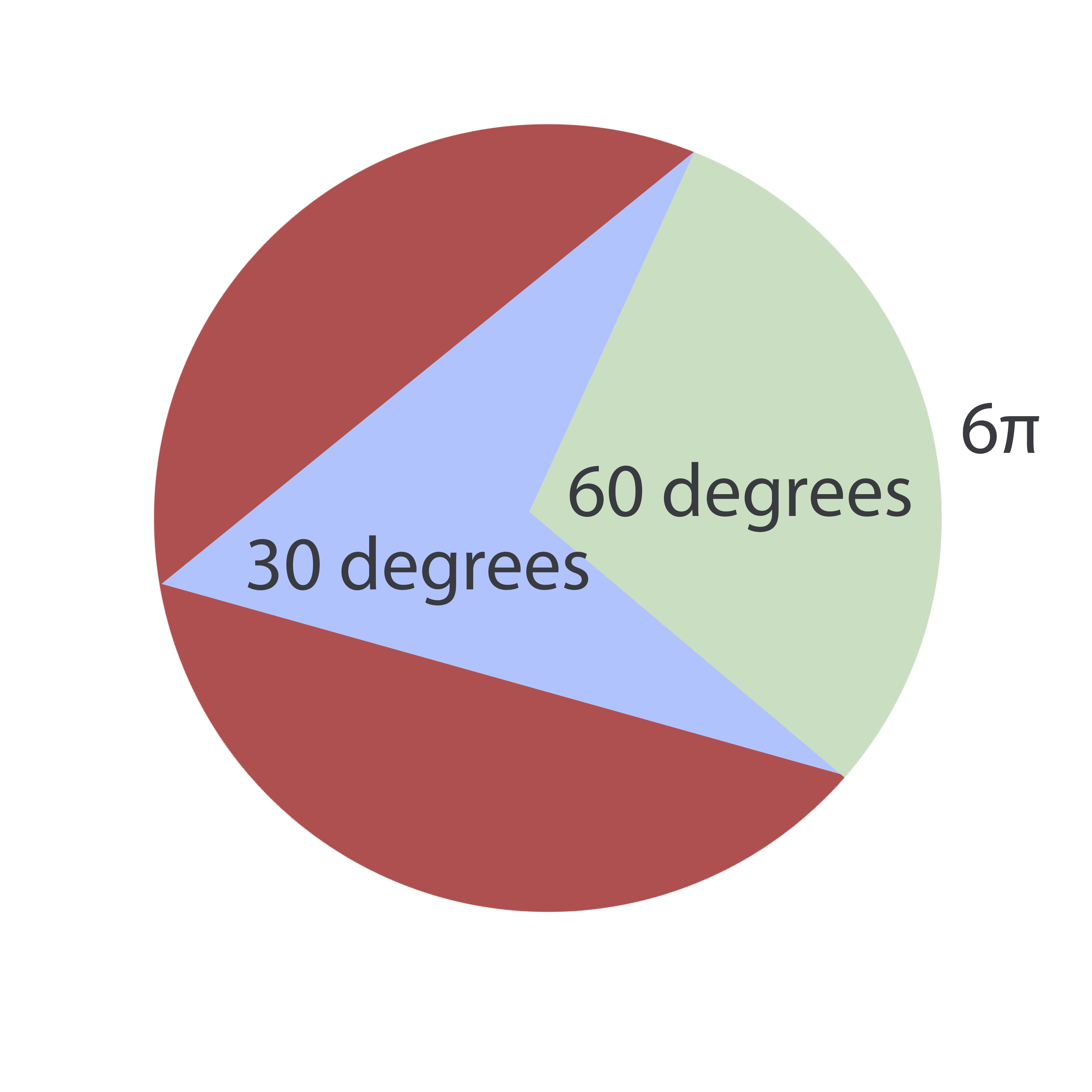Now, the formula for an arc length,, of a circle of a given radius,, and a given angle,is:Since the radius is the unknown, this equation can be rewritten as: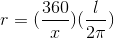Plugging in our values, we find: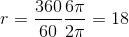### Example Question #3 : How To Find The Percentage Of A Sector From An Angle

A given pizza with a-inch diameter has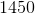calories. A baker cuts the pizza using a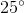angle for each piece. If Susan eats five such pieces, how many calories does she consume? Round to the nearest calorie.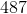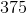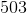Explanation:

To solve this, notice that one piece of pizza comprises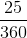of the total pie or (reducing)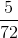of a pie. Now, if Susan buys five slices, she gets: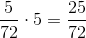of the pie. If the complete pie containscalories, she will then eat the following amount of calories: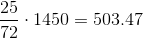Rounding, this iscalories.

### Example Question #1 : How To Find The Angle For A Percentage Of A Circle

An ant begins at the center of a pie with a 12" radius. Walking out to the edge of pie, it then proceeds along the outer edge for a certain distance. At a certain point, it turns back toward the center of the pie and returns to the center point. Its whole trek was 55.3 inches.  What is the approximate size of the angle through which it traveled?

81.53°

149.52°

128.21°

74.76°

91.44°

149.52°

Explanation:

To solve this, we must ascertain the following:

1) The arc length through which the ant traveled.

2) The percentage of the total circumference in light of that arc length.

3) The percentage of 360° proportionate to that arc percentage.

To begin, let's note that the ant travelled 12 + 12 + x inches, where x is the outer arc distance. (It traveled the radius twice, remember); therefore, we know that 24 + x = 55.3 or x = 31.3.

Now, the total circumference of the circle is 2πr or 24π.  The arc is 31.3/24π percent of the total circumference; therefore, the percentage of the angle is 360 * 31.3/24π. Since the answers are approximations, use 3.14 for π. This would be 149.52°.

← Previous 1

Tired of practice problems?

Try live online GRE prep today.